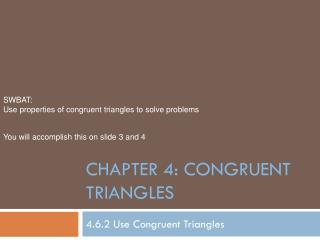DownloadDownload PresentationChapter 4: Congruent Triangles

# Chapter 4: Congruent Triangles

Download Presentation## Chapter 4: Congruent Triangles

- - - - - - - - - - - - - - - - - - - - - - - - - - - E N D - - - - - - - - - - - - - - - - - - - - - - - - - - -
##### Presentation Transcript

1. SWBAT: Use properties of congruent triangles to solve problems You will accomplish this on slide 3 and 4 Chapter 4: Congruent Triangles 4.6.2 Use Congruent Triangles

2. Proofs: More Shapes • We have looked at proofs for 3 sided (triangles) and 4 sided (quadrilaterals (2 x Triangles and 4x Triangles) • Now we need to look at shapes with more sides, there are more combinations of triangles

3. Convex Polygons: Pentagon • Figure ABCDE and Figure VWXYZ are regular pentagons. • prove VY  AC Z E V A D Y C B X W

4. Concave Polygons: • Prove VYX  WYZ • Look at TYZ  UYX, why? • Look at TYU • How would subtraction help? T T U U V W X X Y Y Y Y Z Z

5. Redraw the diagram • As you have seen it might be a good idea to copy the triangles in the diagram into another diagram. • This works for all diagrams, feel free to do transformations, they are congruent.

6. Homework • P. 259 • 6, 12, 13, 14, 20, 21, 33, 40